# APF Imagination Machine

## Listed and emulated in MAME !
Info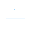(0)
Edit
Upload
### APF Imagination Machine © 1979 APF Electronics, Incorporated.

A combination home video game console and computer system.

### TECHNICAL INFORMATION

1
In basic, pressing FUNCTION and a letter/number will display a basic keyword making it easier to enter programs...
FUNCTION+Q = IF
FUNCTION+W = STEP
FUNCTION+E = STOP
FUNCTION+T = NEXT
FUNCTION+Y = PRINT
FUNCTION+U = END
FUNCTION+I = LIST
FUNCTION+O = REM
FUNCTION+P = USING
FUNCTION+1 = GOSUB
FUNCTION+2 = LET
FUNCTION+3 = DATA
FUNCTION+4 = INPUT
FUNCTION+5 = DIM
FUNCTION+6 = FOR
FUNCTION+7 = RETURN
FUNCTION+8 = THEN
FUNCTION+9 = ON
FUNCTION+0 = GOTO
FUNCTION+- = RESTORE